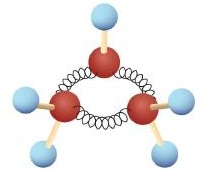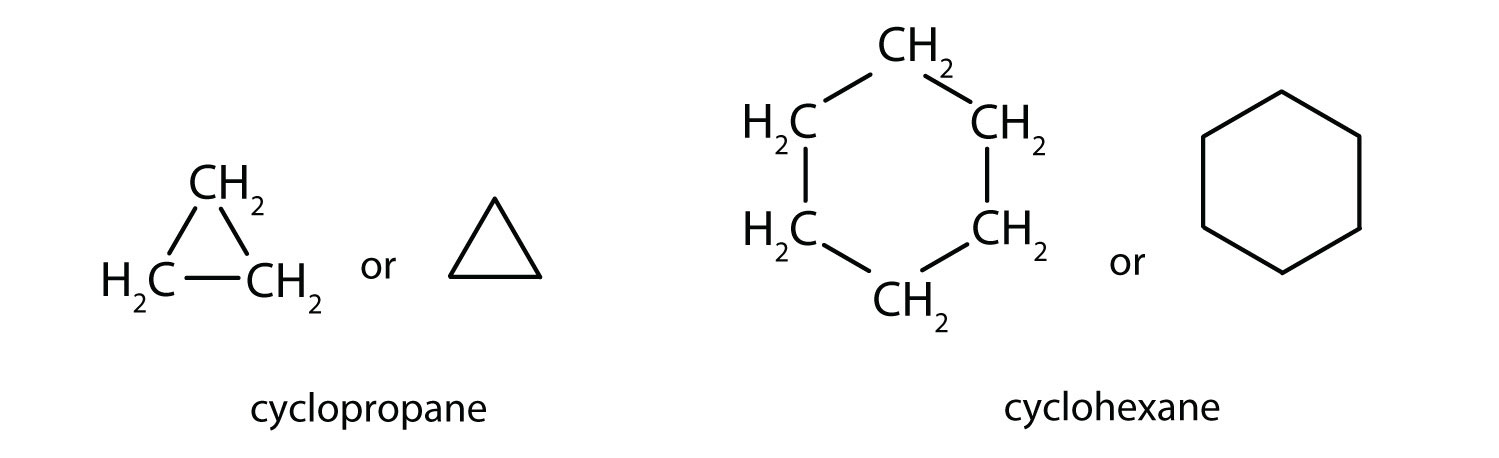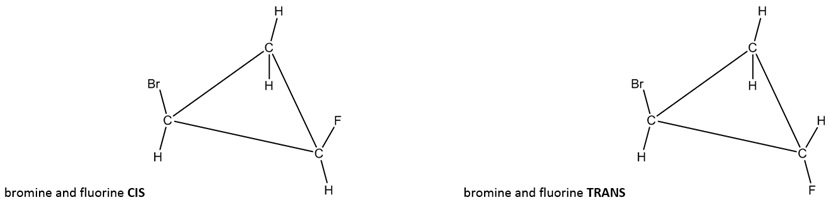# 11.4: Cycloalkanes

$$\newcommand{\vecs}{\overset { \rightharpoonup} {\mathbf{#1}} }$$ $$\newcommand{\vecd}{\overset{-\!-\!\rightharpoonup}{\vphantom{a}\smash {#1}}}$$$$\newcommand{\id}{\mathrm{id}}$$ $$\newcommand{\Span}{\mathrm{span}}$$ $$\newcommand{\kernel}{\mathrm{null}\,}$$ $$\newcommand{\range}{\mathrm{range}\,}$$ $$\newcommand{\RealPart}{\mathrm{Re}}$$ $$\newcommand{\ImaginaryPart}{\mathrm{Im}}$$ $$\newcommand{\Argument}{\mathrm{Arg}}$$ $$\newcommand{\norm}{\| #1 \|}$$ $$\newcommand{\inner}{\langle #1, #2 \rangle}$$ $$\newcommand{\Span}{\mathrm{span}}$$ $$\newcommand{\id}{\mathrm{id}}$$ $$\newcommand{\Span}{\mathrm{span}}$$ $$\newcommand{\kernel}{\mathrm{null}\,}$$ $$\newcommand{\range}{\mathrm{range}\,}$$ $$\newcommand{\RealPart}{\mathrm{Re}}$$ $$\newcommand{\ImaginaryPart}{\mathrm{Im}}$$ $$\newcommand{\Argument}{\mathrm{Arg}}$$ $$\newcommand{\norm}{\| #1 \|}$$ $$\newcommand{\inner}{\langle #1, #2 \rangle}$$ $$\newcommand{\Span}{\mathrm{span}}$$

Skills to Develop

• To draw and name basic cycloalkanes.
• Recognize that alkenes that can exist as geometric isomers and classify isomers as cis or trans.

The hydrocarbons we have encountered so far have been composed of molecules with open-ended chains of carbon atoms. When a chain contains three or more carbon atoms, the atoms can join to form ring or cyclic structures. The simplest of these cyclic hydrocarbons has the formula C3H6. Each carbon atom has two hydrogen atoms attached (Figure $$\PageIndex{1}$$) and is called cyclopropane.Figure $$\PageIndex{1}$$: Ball-and-Spring Model of Cyclopropane. The springs are bent to join the carbon atoms.

## To Your Health: Cyclopropane as an Anesthetic

With its boiling point of −33°C, cyclopropane is a gas at room temperature. It is also a potent, quick-acting anesthetic with few undesirable side effects in the body. It is no longer used in surgery, however, because it forms explosive mixtures with air at nearly all concentrations.

The cycloalkanes—cyclic hydrocarbons with only single bonds—are named by adding the prefix cyclo- to the name of the open-chain compound having the same number of carbon atoms as there are in the ring. Thus the name for the cyclic compound C4H8 is cyclobutane. The carbon atoms in cyclic compounds can be represented by line-angle formulas that result in regular geometric figures. Keep in mind, however, that each corner of the geometric figure represents a carbon atom plus as many hydrogen atoms as needed to give each carbon atom four bonds.Figure $$\PageIndex{2}$$: Condensed structures and line-angle structures of two cycloalkanes

Example $$\PageIndex{1}$$

1. Draw the structure of cyclopentane.

1. Propose a name for this structure.SOLUTION

1. The name cyclopentane indicates a cyclic (cyclo) alkane with five (pent-) carbon atoms. It can be represented as a pentagon.2. The name would be cylcoheptane for this ring of seven carbons (seven-cornered heptagon).

## Cis-Trans Geometric Isomers

To obtain tetrahedral 109.50 bond angles around each carbon in a cyclohexane, most rings bend or "pucker" slightly in three dimenstions. Despite this, it will be easier for us to show all cycloalkanes as planar (flat) here. Every carbon a cylcoalkane must have two bonds to other carbons in the ring, plus two other bonds (to make four bonds for an octet). Of the two bonds that are not a part of the ring, one will point up from the plane of the ring and one will point down.Figure $$\PageIndex{3}$$: Lewis structure of cyclopropane, showing how each carbon has a bond to hydrogen up and down

If two of the hydrogens on different carbons are replaced by other groups, those new groups can make two new geometric isomers. If both groups are pointing in the same direction, both up or both down, it is called cis (Latin derived “on the near side of; on this side of”). If the two groups are pointing in the opposite direction, one up and one down, it is called trans (Latin derived "across from; on the other side of").Figure $$\PageIndex{4}$$: Cis and trans examples of a disubstituted cyclopropane ring (bromine on one carbon and fluorine on another

The above, left image shows a bromine pointing up on one carbon and a fluorine pointing up on another carbon (cis). The above, right image shows a bromine pointing up on one carbon and a fluorine pointing down on another carbon (trans).

Note that if both non-hydrogen groups are bonded to the same carbon of the ring, cis and trans are NOT used because there are not two different ways to make those bonds. (If on the same carbon, one must be up and the other must be down; it is not called trans because there is no cis possiblity.)

When drawing line-angle (skeletal) structures for the rings, the carbons and hydrogens are not shown. To be clear if the non-H groups are up or down, we typically either use vertical lines (up/down) or wedges and dashes. For example, all three images below are different ways to show the same trans molecule (Cl up and CH3 down).Figure $$\PageIndex{5}$$: Three ways to show a trans disubstitured cylcopropane ring (with chlorine on one carbon and CH3 on another carbon)

NOTE: Labeling geometric isomers of cycloalkanes with more than two non-hydrogen substituent groups is more complicated and will not be covered in this text.

## Concept Review Exercises

1. What is the molecular formula of cyclooctane? Also draw a line-angle structure for cylcooctane.

1. C8H16## Key Takeaway

• Many organic compounds have cyclic structures.
• If a cycloalkane ring has two non-H groups on different carbons, two geometric isomers are possible --- cis and trans.

## Exercises

1. Each of the following cylcoalkane rings have two hydrogens substituted for other groups (disubstituted). Label each as cis, trans, or neither.2.